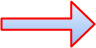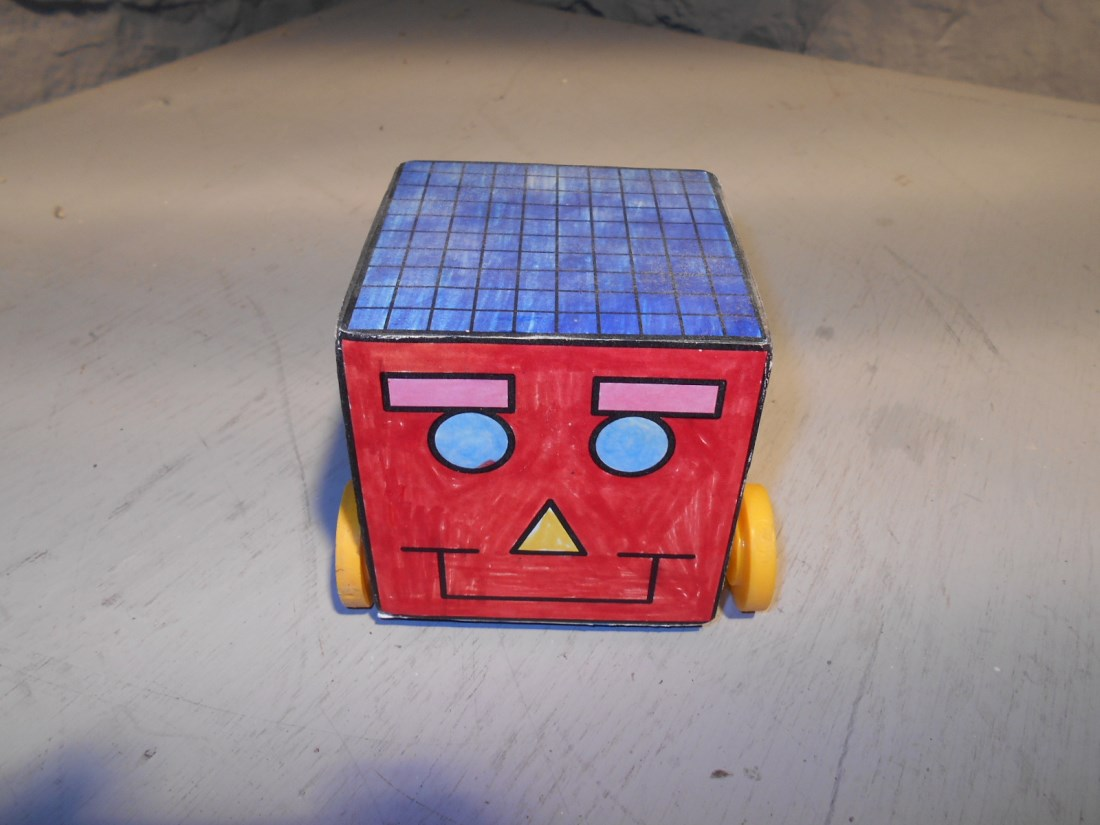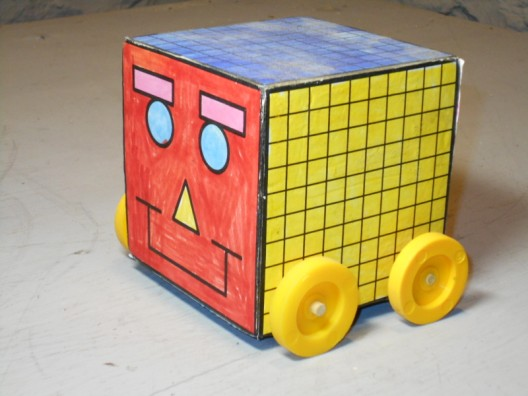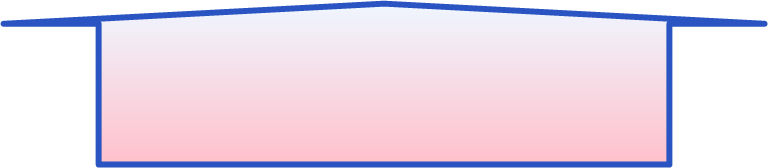Cubee "D"
(d³)
Hello Girls and Boys.
My name is Cubee "D". I am a cube. I have six surfaces, eight vertexes, and twelve edges.Surface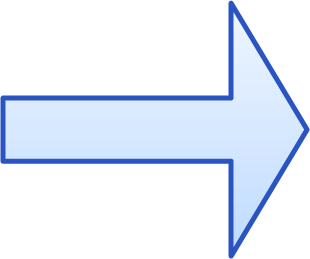Edge
I am also a cubic decimeter. I belong
to the metric family. I am one decimeter long, one decimeter wide and one decimeter in height. I have a short way of writing cubic decimeter;
(dm³)
Vextex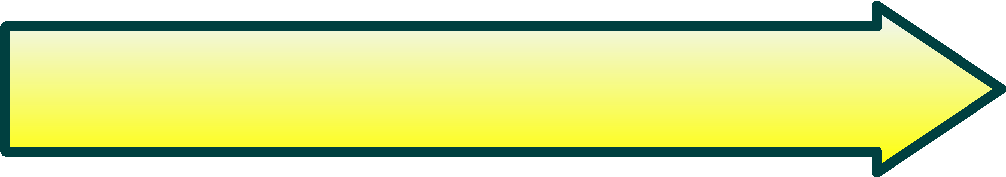Height = one decimeterLength = one decimeterWidth = one decimeter
I can measure:SquaresRectangles
Lines
Circles
Triangles
My friend Ell can measure liquids. Go see what she can do.